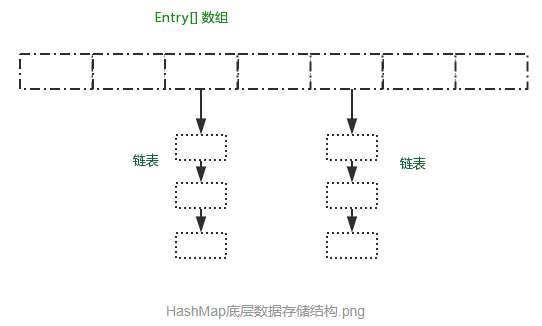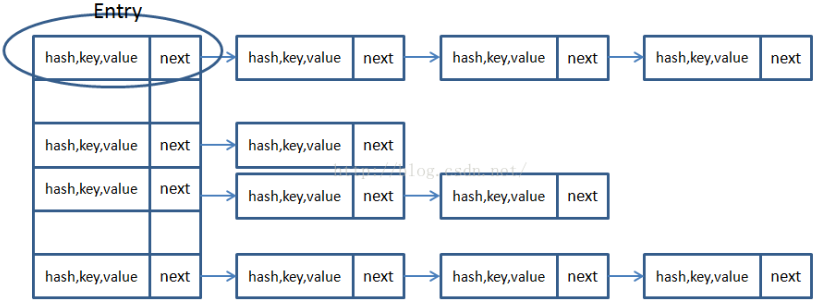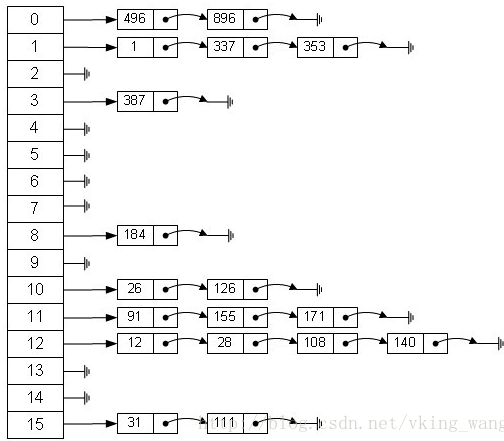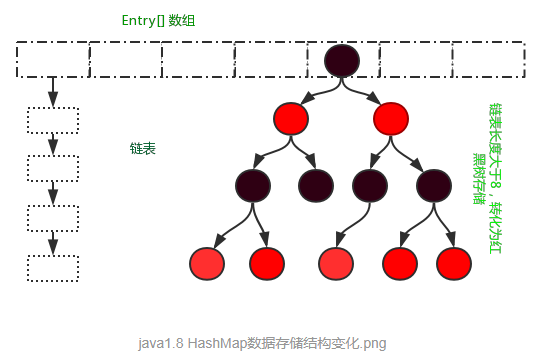# Java集合：HashMap底层实现和原理（源码解析）

## 一、先来熟悉一下我们常用的HashMap:

### 1、概述

HashMap基于Map接口实现，元素以键值对的方式存储，并且允许使用null 建和null　值，　因为key不允许重复，因此只能有一个键为null,另外HashMap不能保证放入元素的顺序，它是无序的，和放入的顺序并不能相同。HashMap是线程不安全的。

### 2、继承关系

public class HashMap<K,V>extends AbstractMap<K,V>
implements Map<K,V>, Cloneable, Serializable 

### 3、基本属性

static final int DEFAULT_INITIAL_CAPACITY = 1 << 4; //默认初始化大小 16
static final float DEFAULT_LOAD_FACTOR = 0.75f;     //负载因子0.75
static final Entry<?,?>[] EMPTY_TABLE = {};         //初始化的默认数组
transient int size;     //HashMap中元素的数量
int threshold;          //判断是否需要调整HashMap的容量  

Note：HashMap的扩容操作是一项很耗时的任务，所以如果能估算Map的容量，最好给它一个默认初始值，避免进行多次扩容。HashMap的线程是不安全的，多线程环境中推荐是ConcurrentHashMap。

## 二、常被问到的HashMap和Hashtable的区别

### 1、线程安全

Hashtable的实现方法里面都添加了synchronized关键字来确保线程同步，因此相对而言HashMap性能会高一些，我们平时使用时若无特殊需求建议使用HashMap，在多线程环境下若使用HashMap需要使用Collections.synchronizedMap()方法来获取一个线程安全的集合。

Note：

Collections.synchronizedMap()实现原理是Collections定义了一个SynchronizedMap的内部类，这个类实现了Map接口，在调用方法时使用synchronized来保证线程同步,当然了实际上操作的还是我们传入的HashMap实例，简单的说就是Collections.synchronizedMap()方法帮我们在操作HashMap时自动添加了synchronized来实现线程同步，类似的其它Collections.synchronizedXX方法也是类似原理。

### 2、针对null的不同

HashMap可以使用null作为key，而Hashtable则不允许null作为key

Note：HashMap以null作为key时，总是存储在table数组的第一个节点上。

### 3、继承结构

HashMap是对Map接口的实现，HashTable实现了Map接口和Dictionary抽象类。

### 4、初始容量与扩容

HashMap的初始容量为16，Hashtable初始容量为11，两者的填充因子默认都是0.75。

HashMap扩容时是当前容量翻倍即:capacity*2，Hashtable扩容时是容量翻倍+1即:capacity*2+1。

### 5、两者计算hash的方法不同

Hashtable计算hash是直接使用key的hashcode对table数组的长度直接进行取模

int hash = key.hashCode();
int index = (hash & 0x7FFFFFFF) % tab.length;

HashMap计算hash对key的hashcode进行了二次hash，以获得更好的散列值，然后对table数组长度取摸。

int hash = hash(key.hashCode());
int i = indexFor(hash, table.length);

static int hash(int h) {
// This function ensures that hashCodes that differ only by
// constant multiples at each bit position have a bounded
// number of collisions (approximately 8 at default load factor).
h ^= (h >>> 20) ^ (h >>> 12);
return h ^ (h >>> 7) ^ (h >>> 4);
}

static int indexFor(int h, int length) {
return h & (length-1);

## 三、HashMap的数据存储结构

### 1、HashMap由数组和链表来实现对数据的存储

HashMap采用Entry数组来存储key-value对，每一个键值对组成了一个Entry实体，Entry类实际上是一个单向的链表结构，它具有Next指针，可以连接下一个Entry实体，以此来解决Hash冲突的问题。HashMap里面实现一个静态内部类Entry，其重要的属性有 hash，key，value，next。

HashMap里面用到链式数据结构的一个概念。上面我们提到过Entry类里面有一个next属性，作用是指向下一个Entry。打个比方， 第一个键值对A进来，通过计算其key的hash得到的index=0，记做:Entry = A。一会后又进来一个键值对B，通过计算其index也等于0，现在怎么办？HashMap会这样做:B.next = A,Entry = B,如果又进来C,index也等于0,那么C.next = B,Entry = C；这样我们发现index=0的地方其实存取了A,B,C三个键值对,他们通过next这个属性链接在一起。所以疑问不用担心。也就是说数组中存储的是最后插入的元素。到这里为止，HashMap的大致实现，我们应该已经清楚了。

public V put(K key, V value) {
if (key == null)
return putForNullKey(value); //null总是放在数组的第一个链表中
int hash = hash(key.hashCode());
int i = indexFor(hash, table.length);
//遍历链表
for (Entry<K,V> e = table[i]; e != null; e = e.next) {
Object k;
//如果key在链表中已存在，则替换为新value
if (e.hash == hash && ((k = e.key) == key || key.equals(k))) {
V oldValue = e.value;
e.value = value;
e.recordAccess(this);
return oldValue;
}
}

modCount++;
addEntry(hash, key, value, i);
return null;
}

void addEntry(int hash, K key, V value, int bucketIndex) {
Entry<K,V> e = table[bucketIndex];
table[bucketIndex] = new Entry<K,V>(hash, key, value, e); //参数e, 是Entry.next
//如果size超过threshold，则扩充table大小。再散列
if (size++ >= threshold)
resize(2 * table.length);
}

## 四、重要方法深度解析

### 1、构造方法

HashMap()    //无参构造方法
HashMap(int initialCapacity)  //指定初始容量的构造方法
HashMap(int initialCapacity, float loadFactor) //指定初始容量和负载因子
HashMap(Map<? extends K,? extends V> m)  //指定集合，转化为HashMap


HashMap提供了四个构造方法，构造方法中 ，依靠第三个方法来执行的，但是前三个方法都没有进行数组的初始化操作，即使调用了构造方法此时存放HaspMap中数组元素的table表长度依旧为0 。在第四个构造方法中调用了inflateTable()方法完成了table的初始化操作，并将m中的元素添加到HashMap中。

### 2、添加方法

public V put(K key, V value) {
if (table == EMPTY_TABLE) { //是否初始化
inflateTable(threshold);
}
if (key == null) //放置在0号位置
return putForNullKey(value);
int hash = hash(key); //计算hash值
int i = indexFor(hash, table.length);  //计算在Entry[]中的存储位置
for (Entry<K,V> e = table[i]; e != null; e = e.next) {
Object k;
if (e.hash == hash && ((k = e.key) == key || key.equals(k))) {
V oldValue = e.value;
e.value = value;
e.recordAccess(this);
return oldValue;
}
}

modCount++;
addEntry(hash, key, value, i); //添加到Map中
return null;
}

### 3、addEntry()

/*
* hash hash值
* key 键值
* value value值
* bucketIndex Entry[]数组中的存储索引
* /
void addEntry(int hash, K key, V value, int bucketIndex) {
if ((size >= threshold) && (null != table[bucketIndex])) {
resize(2 * table.length); //扩容操作，将数据元素重新计算位置后放入newTable中，链表的顺序与之前的顺序相反
hash = (null != key) ? hash(key) : 0;
bucketIndex = indexFor(hash, table.length);
}

createEntry(hash, key, value, bucketIndex);
}
void createEntry(int hash, K key, V value, int bucketIndex) {
Entry<K,V> e = table[bucketIndex];
table[bucketIndex] = new Entry<>(hash, key, value, e);
size++;
}

### 4、获取方法：get

public V get(Object key) {
if (key == null)
//返回table 的value值
return getForNullKey();
Entry<K,V> entry = getEntry(key);

return null == entry ? null : entry.getValue();
}
final Entry<K,V> getEntry(Object key) {
if (size == 0) {
return null;
}

int hash = (key == null) ? 0 : hash(key);
for (Entry<K,V> e = table[indexFor(hash, table.length)];
e != null;
e = e.next) {
Object k;
if (e.hash == hash &&
((k = e.key) == key || (key != null && key.equals(k))))
return e;
}
return null;
}

在get方法中，首先计算hash值，然后调用indexFor()方法得到该key在table中的存储位置，得到该位置的单链表，遍历列表找到key和指定key内容相等的Entry，返回entry.value值。

### 5、删除方法

public V remove(Object key) {
Entry<K,V> e = removeEntryForKey(key);
return (e == null ? null : e.value);
}
final Entry<K,V> removeEntryForKey(Object key) {
if (size == 0) {
return null;
}
int hash = (key == null) ? 0 : hash(key);
int i = indexFor(hash, table.length);
Entry<K,V> prev = table[i];
Entry<K,V> e = prev;

while (e != null) {
Entry<K,V> next = e.next;
Object k;
if (e.hash == hash &&
((k = e.key) == key || (key != null && key.equals(k)))) {
modCount++;
size--;
if (prev == e)
table[i] = next;
else
prev.next = next;
e.recordRemoval(this);
return e;
}
prev = e;
e = next;
}

return e;
}


删除操作，先计算指定key的hash值，然后计算出table中的存储位置，判断当前位置是否Entry实体存在，如果没有直接返回，若当前位置有Entry实体存在，则开始遍历列表。定义了三个Entry引用，分别为pre, e ,next。 在循环遍历的过程中，首先判断pre 和 e 是否相等，若相等表明，table的当前位置只有一个元素，直接将table[i] = next = null 。若形成了pre -> e -> next 的连接关系，判断e的key是否和指定的key 相等，若相等则让pre -> next ,e 失去引用。

### 6、containsKey

public boolean containsKey(Object key) {
return getEntry(key) != null;
}
final Entry<K,V> getEntry(Object key) {
int hash = (key == null) ? 0 : hash(key.hashCode());
for (Entry<K,V> e = table[indexFor(hash, table.length)];
e != null;
e = e.next) {
Object k;
if (e.hash == hash &&
((k = e.key) == key || (key != null && key.equals(k))))
return e;
}
return null;
}

containsKey方法是先计算hash然后使用hash和table.length取摸得到index值，遍历table[index]元素查找是否包含key相同的值。

### 7、containsValue

public boolean containsValue(Object value) {
if (value == null)
return containsNullValue();

Entry[] tab = table;
for (int i = 0; i < tab.length ; i++)
for (Entry e = tab[i] ; e != null ; e = e.next)
if (value.equals(e.value))
return true;
return false;
}

containsValue方法就比较粗暴了，就是直接遍历所有元素直到找到value，由此可见HashMap的containsValue方法本质上和普通数组和list的contains方法没什么区别，你别指望它会像containsKey那么高效。

## 五、JDK 1.8的 改变

### 1、HashMap采用数组+链表+红黑树实现。### 2、put方法简单解析：

public V put(K key, V value) {
//调用putVal()方法完成
return putVal(hash(key), key, value, false, true);
}

final V putVal(int hash, K key, V value, boolean onlyIfAbsent,
boolean evict) {
Node<K,V>[] tab; Node<K,V> p; int n, i;
//判断table是否初始化，否则初始化操作
if ((tab = table) == null || (n = tab.length) == 0)
n = (tab = resize()).length;
//计算存储的索引位置，如果没有元素，直接赋值
if ((p = tab[i = (n - 1) & hash]) == null)
tab[i] = newNode(hash, key, value, null);
else {
Node<K,V> e; K k;
//节点若已经存在，执行赋值操作
if (p.hash == hash &&
((k = p.key) == key || (key != null && key.equals(k))))
e = p;
//判断链表是否是红黑树
else if (p instanceof TreeNode)
//红黑树对象操作
e = ((TreeNode<K,V>)p).putTreeVal(this, tab, hash, key, value);
else {
//为链表，
for (int binCount = 0; ; ++binCount) {
if ((e = p.next) == null) {
p.next = newNode(hash, key, value, null);
//链表长度8，将链表转化为红黑树存储
if (binCount >= TREEIFY_THRESHOLD - 1) // -1 for 1st
treeifyBin(tab, hash);
break;
}
//key存在，直接覆盖
if (e.hash == hash &&
((k = e.key) == key || (key != null && key.equals(k))))
break;
p = e;
}
}
if (e != null) { // existing mapping for key
V oldValue = e.value;
if (!onlyIfAbsent || oldValue == null)
e.value = value;
afterNodeAccess(e);
return oldValue;
}
}
//记录修改次数
++modCount;
//判断是否需要扩容
if (++size > threshold)
resize();
//空操作
afterNodeInsertion(evict);
return null;
}

如果存在key节点，返回旧值，如果不存在则返回Null。

03-2218307-037719
04-11172
06-059万+
11-20668
02-28440
03-09824
12-12215
05-19319
07-17286
08-19823
04-19224
05-193361
05-04427
01-222059
12-2543
08-263168
02-25816
04-02144
©️2020 CSDN 皮肤主题: 游动-白 设计师:白松林